Fitting AlgorithmNext: An Example: The Up: Synthetic Rotation Curve Previous: SpectraPSF and

Fitting Algorithm

Non-linear model fitting can be approached in two ways. One involves a gradient method such as the Levenberg-Marquardt method which uses the gradient and a second derivative matrix to quickly find the local minimum nearest to the starting point. The other involves searching for the absolute minimum by taking steps with Monte-Carlo generated sizes and directions through parameter space. A variant of this approach called the Metropolis algorithm [\protect\astronciteSaha and Williams1994][\protect\astroncitePress et al.1986] was used. It MonteCarlo samples parameter space with a sampling density proportional to the likelihood. It has less chances of getting trapped in the first local minimum it encounters. Confidence intervals can be directly calculated as the same time the algorithm is looking for the best parameter values.

Let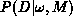be the probability distribution that a set of data D will be collected given a model M with a set of parameter values.is not in itself interesting because the parameter values are not known. Using Bayes' theorem, it is possible to invertto get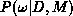, the probability distribution thatwill be observed given a model and a set of data. This distribution may be used to define best parameter values and suitable confidence intervals on those values. Bayes' theorem here can be expressed as: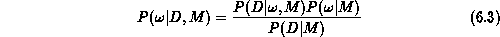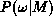is called the prior probability of the parameters, and it represents whatever is known (if anything is) about parameter values before taking any data.is called the likelihood,is called the posterior probability distribution and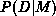is just a normalizing factor called the global likelihood. Equationsimply states thatis proportional to the likelihood. For Gaussian noise, the likelihood that N measurements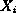are consistent with pure noise is given by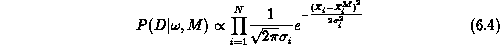The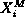's are the predictions of the model M for the set of parameter values. Carrying out the product in equation,becomes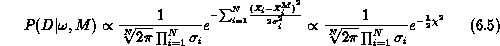In read-out and sky noise limited CCD images, the photon noise due to the galaxy signal is negligible, and the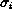's are all equal to the background noise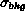, so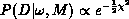. The background noisewas determined for each spectrum by computing image statistics in four regions at the corners of the spectrum.

The Metropolis algorithm starts with some set of parameter valuesand the associated. It then picks a possible change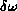in the parameters and computes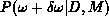. If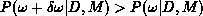, then the changeis accepted. If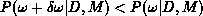, then the changeis accepted only some fraction/of the time. After hundreds of such iterations, the distribution of accepted values ofwill converge toprovided that all possibleare eventually accessible. Parameter space is thus sampled with a density proportional to the likelihood. The trial changesare chosen at random. If they are too small (i.e. the parameter search is too ``cold''), then all iterations will accept changes. On the other hand, if the search is too ``hot'', then none of the iterations will accept changes. It is usual to regulate the size of the steps (i.e. the temperature of the search) so that half the iterations accept changes.

Temperature control is thus an important element of the Metropolis. The temperature control scheme must allow the search to sample the largest volume in parameter space with the lowest possible number of steps. In the current implementation of the Metropolis, it starts with a n-dimensional volume centered on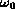given by V=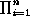T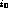where Tis the initial temperature on the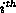parameter. Parameter values are generated using=+Twhereis a random number between1 and 1. If the first lower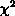value is found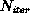iterations after the beginning of the search, then one would expect that this region would have a characteristic size V = V/. In order to match the search temperature to the size of that lowerregion, the temperature of each parameter is then reduced according to T= T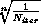. After this initial cooling, the search continues. The ratio of the number of acceptedover the number of trialis computed after every ten trials. If less than half the changes have been accepted, then the parameter temperatures are all decreased by 30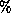. If not, then the parameter temperatures are all increased by 30.

The best parameter values were chosen to be the median's of. These median values were obtained by simply sorting out the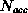acceptedvalues and taking the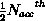value. The 0.16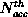and 0.84values were used as the lower and upper bounds of the 68confidence intervals.Next: An Example: The Up: Synthetic Rotation Curve Previous: SpectraPSF and

Luc Simard
Mon Sep 2 12:37:40 PDT 1996